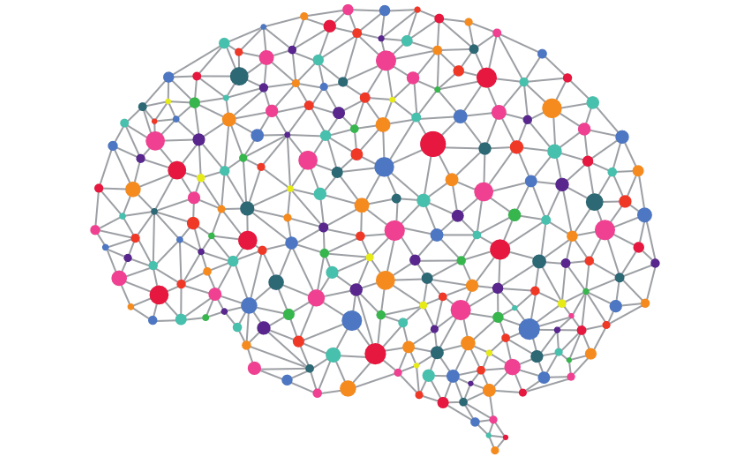# Classification Rules in Machine Learning

Classification rules represent knowledge in an if-else format. These types of rules involve the terms antecedent and consequent. The antecedent is the before and consequent is after. For example, I may have the following rule.

If the students studies 5 hours a week then they will pass the class with an A

This simple rule can be broken down into the following antecedent and consequent.

• Antecedent–If the student studies 5 hours a week
• Consequent-then they will pass the class with an A

The antecedent determines if the consequent takes place. For example, thestudent must study 5 hours a week to get an A. This is the rule in this particular context.

This post will further explain the characteristics and traits of classification rules.

Classification Rules and Decision Trees

Classification rules are developed on current data to make decisions about future actions. They are highly similar to the more common decision trees. The primary difference is that decision trees involve a complex step-by-step process to make a decision.

Classification rules are stand-alone rules that are abstracted from a process. To appreciate a classification rule you do not need to be familiar with the process that created it. While with decision trees you do need to be familiar with the process that generated the decision.

One catch with classification rules in machine learning is that the majority of the variables need to be nominal in nature. As such, classification rules are not as useful for large amounts of numeric variables. This is not a problem with decision trees.

The Algorithm

Classification rules use algorithms that employ a separate and conquer heuristic. What this means is that the algorithm will try to separate the data into smaller and smaller subset by generating enough rules to make homogeneous subsets. The goal is always to separate the examples in the data set into subgroups that have similar characteristics.

Common algorithms used in classification rules include the One Rule Algorithm and the RIPPER Algorithm. The One Rule Algorithm analyzes data and generates one all-encompassing rule. This algorithm works by finding the single rule that contains the less amount of error. Despite its simplicity, it is surprisingly accurate.

The RIPPER algorithm grows as many rules as possible. When a rule begins to become so complex that in no longer helps to purify the various groups the rule is pruned or the part of the rule that is not beneficial is removed. This process of growing and pruning rules is continued until there is no further benefit.

RIPPER algorithm rules are more complex than One Rule Algorithm. This allows for the development of complex models. The drawback is that the rules can become too complex to make practical sense.

Conclusion

Classification rules are a useful way to develop clear principles as found in the data. The advantage of such an approach is simplicity. However, numeric data is harder to use when trying to develop such rules.